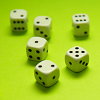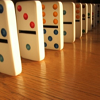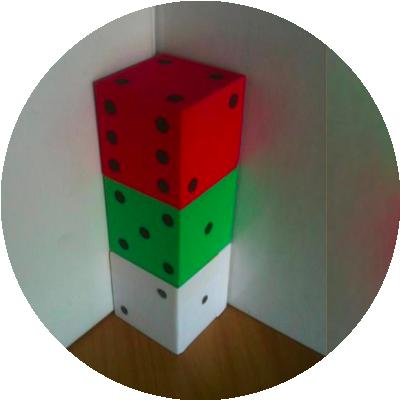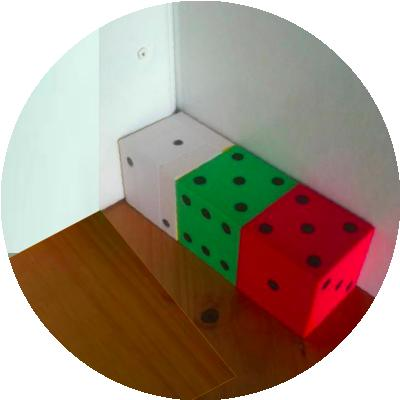#### You may also like### Consecutive Numbers

An investigation involving adding and subtracting sets of consecutive numbers. Lots to find out, lots to explore.### Roll These Dice

Roll two red dice and a green dice. Add the two numbers on the red dice and take away the number on the green. What are all the different possible answers?### Domino Square

Use the 'double-3 down' dominoes to make a square so that each side has eight dots.

# Dice in a Corner

##### Age 7 to 11Challenge LevelThree dice are sitting in the corner with the simple rule that where two faces touch they must be the same numbers.
So, in the first picture above there are $3$'s at the bottom of the red dice and on the top of the middle green and there are $4$'s on the bottom of the green dice and the top of the white dice. The numbers on the seven faces that can be seen are then added and make $21$.

In the second picture above there are $4$'s at the left of the red dice and on the right of the green dice and there are $3$'s on the left of the green dice and the right of the white dice. The numbers on the seven faces that can be seen are then added and make $23$.

Use your own dice (you could use two or three or more...)
Now for a challenge -  arrange dice (using at least $2$ and up to as many as you like) in a line in the corner, so that the faces you can see add up to $18$ in as many ways as possible.